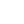# Residual Network

A "fake" network constructed from real network considering the flow in two ways, remaining flow capacity, and current flow capacity which allows to cancel.

# Augmenting path

Path from s to t in residual network

# Residual capacity

The maximum amount of net flow that we can ship along the edges of an augmenting path p, it can be called as bottleneck. Residual capacity on edge(u, v) = real capacity from u to v - used flow from u to v = c(u, v) - f(u, v) Residual capacity on path(u -> v -> s) = min(cf(u,v), cf(v, s))

# Ford-Fulkerson algorithm

```For each edge(u ,v)
f[u, v] = 0 // no flow at start
f[v, u] = 0

while there exist a augmenting path p from s to t
find cf(p) = residual capacity(bottleneck) on the agumenting path
for each edge(u, v) on the agumenting path
f[u, v] += f[u, v] + cf(p)   // we used cf(p) capacity on that tunnel
f[v, u] += f[v, u] - cf(p)   // to be redraw, when you compute cf(v, u) =  c[v, u] - (- xxx) = c[v, u] + xxxx
```

# The Edmonds-Karp algorithm

Use breath first search to find a path

# Maximum bipartite matching:

Link s to t wth two lines, each line weighted 1